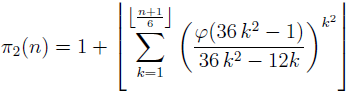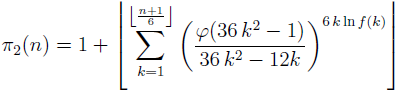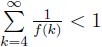# Note on some explicit formulae for twin prime counting function

Notes on Number Theory and Discrete Mathematics, ISSN 1310-5132
Volume 19, 2013, Number 2, Pages 43—48

## Details

### Authors and affiliations

Mladen Vassilev-Missana5 V. Hugo Str., 1124 Sofia, Bulgaria

### Abstract

In the paper for any integer n ≥ 5 the validity of the formula(where π2 denotes the twin prime counting function and φ is Euler’s totient function) is established. Also for any integer n ≥ 5 the formula(where f is arbitrary artihmetic function with strictly positive values satisfying the conditionis proved.

### Keywords

• Prime number (prime)
• Twin primes
• Twin prime counting function
• Arithmetic function

• 11A25
• 11A41

### References

1. Ribenboim, P. The New Book of Prime Number Records (3rd Edition), Springer-Verlag, New York, 1996.
2. Vassilev-Missana, M. Some new formulae for the twin prime counting function π2, Notes on Number Theory and Discrete Mathematics, Vol 7, 2001, No. 1, 10–14.

## Cite this paper

APA

Vassilev-Missana, M. (2013). Note on some explicit formulae for twin prime counting function. Notes on Number Theory and Discrete Mathematics, 19(2), 43-48.

Chicago

Vassilev-Missana, Mladen. “Note on Some Explicit Formulae for Twin Prime Counting Function.” Notes on Number Theory and Discrete Mathematics 19, no. 2 (2013): 43-48.

MLA

Vassilev-Missana, Mladen. “Note on Some Explicit Formulae for Twin Prime Counting Function.” Notes on Number Theory and Discrete Mathematics 19.2 (2013): 43-48. Print.